# How to use the DATEVALUE Function in Excel

In this article,  we will learn how to use the DATEVALUE function in excel.
DATEVALUE function & TIMEVALUE function in excel are very useful function when processing Date & Time data in excel. Date value as text cannot be processed as date in excel. Excel considers date as a serial number, So any date value in a cell which infact has to be in number format to be readable by excel.
While processing date values in excel having large data. First thing recommended is to convert date_text to serial number using DATEVALUE function.
DATEVALUE function convert date as text to date as serial number.
Syntax:

=DATEVALUE(date_text)

date_text : date in text. Date as number will return #VALUE error by the function.
Let’s see some examples using the DATEVALUE function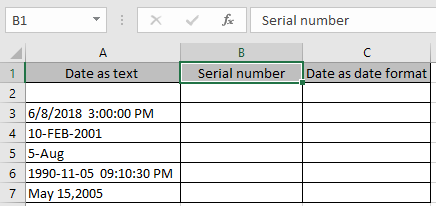Here we have a Date value as text and we need to get these values in serial number which is readable by the excel.
For first cell we will provide the date_text to the function directly instead of giving cell reference.
Use the formula

=DATEVALUE( "6/8/2018" )

Here datevalue is provided as a string to the function.
Press Enter.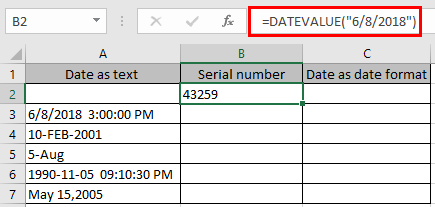Now for using cell reference as an input to the function

Use the formula:

=DATEVALUE(A3)

Here A3 is cell reference to the function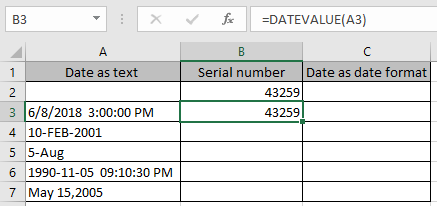The function returns the serial number for the date in the number format.
Now copy the formula to other cells using the shortcut Ctrl+ D.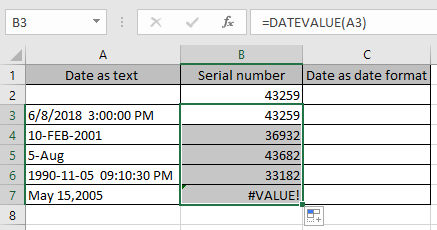These returned serial number by the function is readable by excel as dates. The function returns the #VALUE error if the date is not in text format. The function doesn’t consider already date format value.

IF you need to see these dates in date format. Select the dates and using the format cell option as shown in the below image.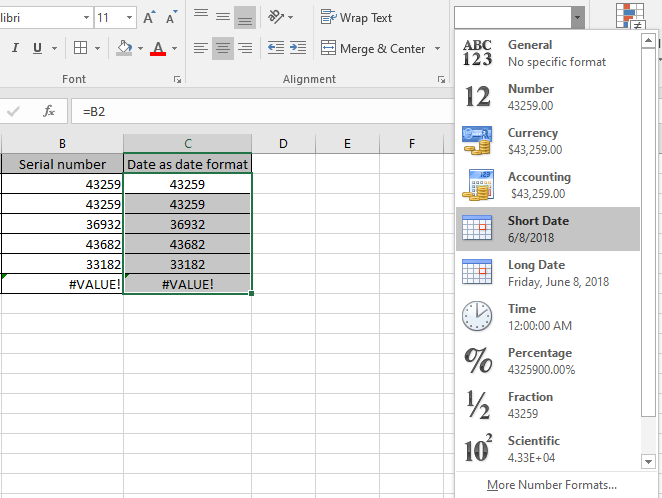As we select the short date option from the drop down result, We get the dates as date format which is also readable by Excel.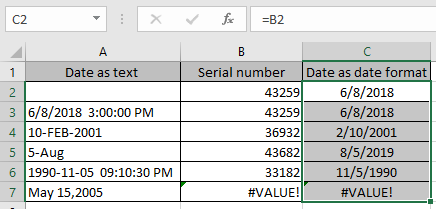As you can we have dates as required.

Hope you understood how to extract date and time from combined date & time in a cell in Excel 2016. Find more articles on Date and Time here. Please share your query below in the comment box. We will assist you.

Related Articles

Excel LOG10 function

How to use the IMEXP Function in Excel

How to use the IMCONJUGATE Function in Excel

How to use the IMARGUMENT Function in Excel

Popular Articles

Edit a dropdown list

If with conditional formatting

If with wildcards

Vlookup by date

Terms and Conditions of use

The applications/code on this site are distributed as is and without warranties or liability. In no event shall the owner of the copyrights, or the authors of the applications/code be liable for any loss of profit, any problems or any damage resulting from the use or evaluation of the applications/code.